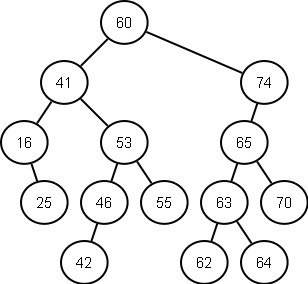Thursday, June 13, 2013

Lowest Common Ancestor in a Binary Search Tree

For the LCA in a simple binary tree go here.

Finding the lowest common ancestor (LCA) in a binary search tree may be easy, but coming up with an elegant and quick solution may take some thought.

A binary search tree is a ordered binary tree where the nodes on the left are always less than the root node and the nodes on the right are always larger than the root node.

Let's use the tree below as an example.Fig.1 - Simple Binary Search Tree

Now keeping in mind that property that makes the binary search tree different that a binary tree we can come up with something that is faster than finding the LCA for a simple binary tree, precisely we'll make it O(h) - where h is the height of the tree. This means that in the tree above it will take maximum 5 iterations to find the LCA.

Let's say we're looking for the nodes 62 and 70.
We take the maximum of these two and compare it to the root, if the maximum is less than the root then our values are in the left branch, and we can forget about the right branch at all. Again, we can do this because of the binary search tree property that all the nodes on the left of the root are less than the root. Since the maximum of our two numbers is not less than the root we can move to the second case.
Now, we take the minimum of those two and compare it with the root. If the minimum is greater than the root, then we go on with the right branch. You may ask: why minimum (or maximum) ? The answer is that the nodes we're looking for might be each other's parent. So if our comparison would not be greater than the root, we would go to our third case which i'll take note later. Since this is the case the minimum of these two is 62 and it is greater the the root - we go further to 74.
We keep doing our previous conditions, until both of them don't succeed:
- max is less than 74, we go on the left to 65
- max is not less than 65, then is min greater than 65 - not even that!
Here we go to our third case: if both previous cases failed, this could not mean anything but that the root is the LCA of nodes we're looking for, which is 65.

Again in code this is nothing but a binary search:

Note:
- Math.max and Math.min = determines the maximum and minimum of two numbers
- In a balanced binary search tree h = log n, so then we have O(log n)
For the node representation we'll use the following class: